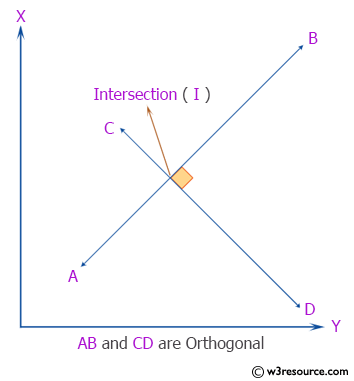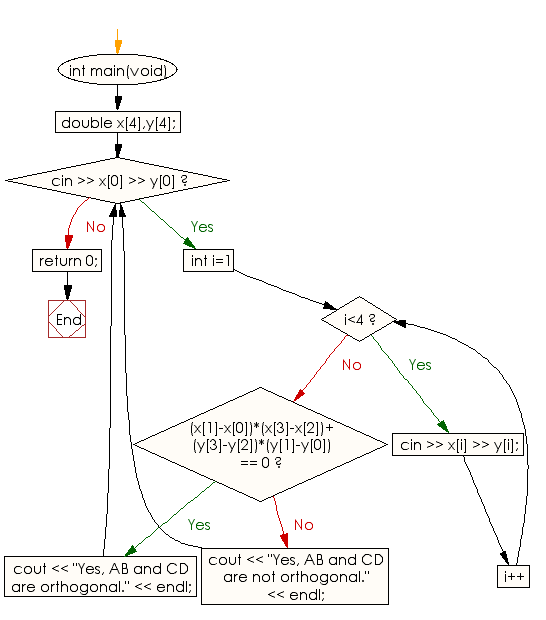﻿ C++ : Check 2 straight lines AB and CD are orthogonal or not

# C++ Exercises: Check whether two straight lines AB and CD are orthogonal or not

## C++ Basic: Exercise-77 with Solution

There are four different points on a plane: A(x1, y1), B(x2, y2), C(x3, y3) and D(x4, y4).
Write a C++ program to check whether two straight lines AB and CD are orthogonal or not.
Input:
0 6
5 6
3 8
3 2
Output:
yes

Pictorial Presentation:Sample Solution:

C++ Code :

``````#include <iostream>
using namespace std;
int main(void)
{
double x,y;
while(cin >> x >> y){
for(int i=1; i<4; i++){
cin >> x[i] >> y[i];
}
if( (x-x)*(x-x)+(y-y)*(y-y) == 0 )
{
cout << "Yes, AB and CD are orthogonal." << endl;
}
else
{
cout << "Yes, AB and CD are not orthogonal." << endl;
}
}
return 0;
}
``````

Sample Output:

```Input number: 0 6
5 6
3 8
3 2
Yes, AB and CD are orthogonal.
```

Flowchart:C++ Code Editor:

What is the difficulty level of this exercise?

﻿

## C++ Programming: Tips of the Day

Why is there no std::stou?

The most pat answer would be that the C library has no corresponding "strtou", and the C++11 string functions are all just thinly veiled wrappers around the C library functions: The std::sto* functions mirror strto*, and the std::to_string functions use sprintf.

Ref: https://bit.ly/3wtz2qA

We are closing our Disqus commenting system for some maintenanace issues. You may write to us at reach[at]yahoo[dot]com or visit us at Facebook